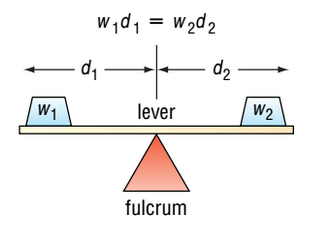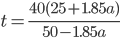### Day 65 - Rational Applications - 11.20.14

 UpdatesUnit 4 Test11/21Summative Exam 211/25Bell RingerWhen two objects are balanced on a lever, their distances from the fulcrum are inversely proportional to their weights. The greater the weight, the less distance it should be from the fulcrum in order to maintain balance. If an 10-kg mass is placed 2.0 meters from the fulcrum, how far should a 40-kg mass be placed from the fulcrum in order to balance the lever?0.5 m2 m1 mnone of the aboveThe formularelates the time t in minutes that it takes to cook an average-size potato in an oven that is at an altitude of a thousands of feet. Calculate the time it takes to cook a potato at an altitude of 3000 feet.40.5 min27.5 min-40.5 min18.9 minIt took 309 days for the Mars Global Surveyor to travel 466,000,000 miles from Earth to Mars. What was the speed of the spacecraft in miles per hour? Round to the nearest 1 decimal place.60,000 mi/hour62,837.1 mi/hour1,508,090.6 mi/hour62,837.1 mi/daynone of the aboveWhile traveling in Canada, I bought some gifts to bring home. I bought three t-shirts that cost \$25.95 (Canadian). If the exchange rate at the time was 1 US dollar for 1.37 Canadian dollars, how much did I spend in US dollars?\$35.55\$18.94\$25.95none of the aboveReviewPrerequisitesDividing by 0Rational NumbersNumerator and DenominatorPolynomialsRational FunctionsPolynomial / PolynomialExcluded ValuesGraphs at Excluded ValuesSimplifying Rational ExpressionsMultiplying/Dividing Rational ExpressionsUnit Conversions (Dimensional Analysis)Dividing PolynomialsLong Division of PolynomialsAdding/Subtracting Rational ExpressionsMixed Expressions and Complex FractionsSolving Rational EquationsVariationDirectInverseLessonExit TicketPosted on board at end of block. Lesson Objective(s)How are direct and inverse variations related?How can direct and inverse variation problems be solved?Standard(s)Solving Rational Equations - 12.9CC.9-12.A.CED.1 Create equations that describe numbers or relationship. Create equations and inequalities in one variable and use them to solve problems. Include equations arising from linear and quadratic functions, and simple rational and exponential functions.CC.9-12.A.REI.11 Represent and solve equations and inequalities graphically. Explain why the x-coordinates of the points where the graphs of the equations y = f(x) and y = g(x) intersect are the solutions of the equation f(x) = g(x); find the solutions approximately, e.g., using technology to graph the functions, make tables of values, or find successive approximations. Include cases where f(x) and/or g(x) are linear, polynomial, rational, absolute value, exponential, and logarithmic functions.CC.9-12.A.REI.2 Understand solving equations as a process of reasoning and explain the reasoning. Solve simple rational and radical equations in one variable, and give examples showing how extraneous solutions may arise.Dividing Polynomials - 12.5CC.9-12.A.APR.6 Rewrite rational expressions. Rewrite simple rational expressions in different forms; write a(x)/b(x) in the form q(x) + r(x)/b(x), where a(x), b(x), q(x), and r(x) are polynomials with the degree of r(x) less than the degree of b(x), using inspection, long division, or, for the more complicated examples, a computer algebra system.Mathematical Practice(s)#1 - Make sense of problems and persevere in solving them#2 - Reason abstractly and quantitatively#4 - Model with mathematics#5 - Use appropriate tools strategically#7 - Look for and make use of structurePast CheckpointsRational ExpressionsCheckpointsSimplifying Rational ExpressionsCheckpointsMultiplying/Dividing Rational ExpressionsCheckpointsDividing PolynomialsCheckpointsAdding/Subtracting Rational ExpressionsCheckpointsMixed Expressions and Complex FractionsCheckpointsSolving Rational EquationsCheckpointsVariationCheckpoints